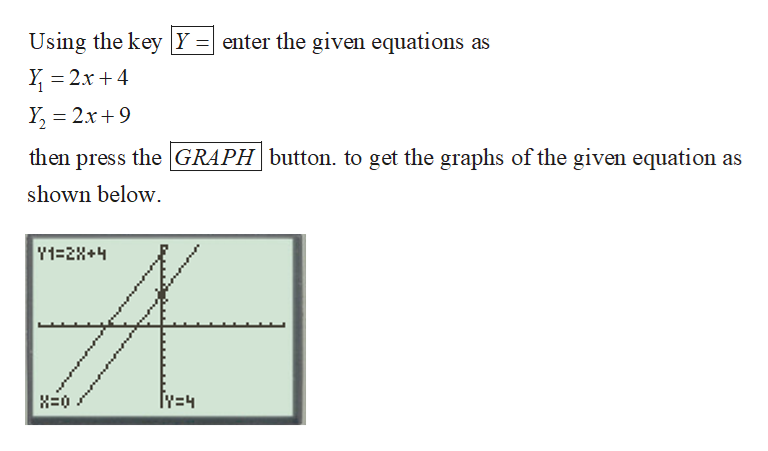Question
1 views
check_circle

Step 1

In order to solve the given system of equation, turn o...help_outlineImage TranscriptioncloseUsing the key Y = enter the given equations as Y, = 2x + 4 Y, = 2x+9 then press the GRAPH button. to get the graphs of the given equation as shown below. Y1=2X+4 Iy=4 fullscreen

### Want to see the full answer?

See Solution

#### Want to see this answer and more?

Solutions are written by subject experts who are available 24/7. Questions are typically answered within 1 hour.*

See Solution
*Response times may vary by subject and question.
Tagged in

### Math